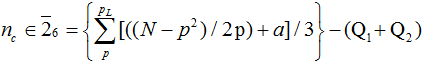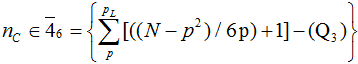# Volume 10, 2004, Number 3

Volume 10Number 1Number 2 ▷ Number 3 ▷ Number 4

On meet and join matrices on A-sets and related sets
Original research paper. Pages 57—67
Ismo Korkee
Full paper (293 Kb) | Abstract

Let (P, ∧) be a meet-semilattice and let S = {x1, x2, …, xn} be a subset of P. We say that S is an A-set if A = {xixj | xixj} is a chain. For example, chains and a-sets (with A = {a}) are known trivial A-sets. The meet matrix (S)f on S with respect to a function f : PC is defined as ((S)f)ij = f(xixj).

We present a recursive structure theorem for meet matrices on A-sets and thus obtain a recursive formula for det(S)f and for (S)f−1 on A-sets. The recursive formulae also yield explicit formulae, e.g. the known determinant and inverse formulae on chains and a-sets. We also present the dual forms of our results, i.e. the determinant formulae and the inverse formulae for join matrices on join-semilattices. Finally, we suggest how our results can be generalized to more complicated cases.

As special cases these results hold also for GCD and LCM matrices and for their unitary analogies GCUD and LCUM matrices.

Relationship between a natural number and its digital product
Original research paper. Pages 68—71
Laurențiu Panaitopol
Full paper (134 Kb) | Abstract

For the integer number n ≥ 1 written in the basis b ≥ 2, we denote by sb(n), pb(n) and db(n) the sum, the product and the number of its digits, respectively. There are a series of more recent or older papers concerning the relationship between these numbers. For instance, one proves in {2} that, if b ≥ 3, then for infinitely many numbers m there exists n with db(n) = m and sb(n) = m, and this property fails also for infinitely many values of m.

Euler’s prime equation within the composite grid of the modular ring Z6
Original research paper. Pages 72—76
J. V. Leyendekkers and A. G. Shannon
Full paper (39 Kb) | Abstract

The extended Euler-prime-generating equation is shown to be compatible with the composite grid of the modular ring Z6. Invalid values of the variable x (those x values which yield composites) follow regular sequences within the grid and have associated couples within the modular ring which are linked to the prime values.

Using integer structure to calculate the number of primes in a given interval
Original research paper. Pages 77—83
J. V. Leyendekkers and A. G. Shannon
Full paper (111 Kb) | Abstract

With the exception of 2 and 3, primes are only found in two classes of the modular ring Z6. The rows of the tabular display of this ring which con­tain composites in these two classes are given by R = R0+ pt, t = 0, 1, 2, 3, …, in which R0 is a function of p that is class specific. The number of composites, nc, in the two classes can be calculated from:(a = 1 or 2 depending on the class of p),where pL is the prime less than and closest to √N. The Qi terms are quantities which arise from the characteristics of the factors of the composites. Subtraction of nc from the total integers in each class yields the number of primes for that class, and hence the total number of primes in the interval.

Remarks on the 46-th Smarandache’s problem
Original research paper. Pages 84—88
Mladen V. Vassilev-Missana and Krassimir T. Atanassov
Full paper (1577 Kb)

Volume 10Number 1Number 2 ▷ Number 3 ▷ Number 4